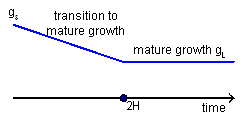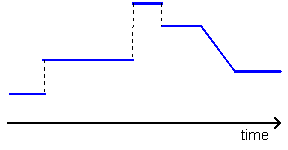### Why should I choose AnalystNotes?

AnalystNotes specializes in helping candidates pass. Period.

##### Subject 7. Multistage Dividend Discount Models
Supernormal growth cannot continue forever. At some point competitors will be attracted by extraordinary profitability and growth will decrease. Most analysts assume that the growth rate of a company stabilizes at some point. The stock value at the beginning of this mature stage is called the terminal value. It can be determined either using the Gordon growth model or by multiplying the company's earnings or sales forecast by the benchmark value of a comparative multiple.

Example

MedSolution is a large pharmaceutical company that is currently in the transitional stage of its business cycle. The company is losing its business to the manufacturers of generic drugs, because a patent on one of its major products expired last year. The dividends growth of MedSolution is expected to decelerate in the next several years and stabilize at 3% after 2007. The dividend for the year 2007 is expected to be \$21. The required rate of return on MedSolution's stock is currently 10% and is not expected to change in the future. The leading price-to-cash flows ratio is expected to be 2.4 at the beginning of 2008. The cash flows will be about \$125. Calculate MedSolution's terminal value using both the GGM and the price multiples approach.

Solution

Using the Gordon growth model we find the value at the beginning of 2008: VTERM = D2007 (1 + g) / (r - g) = 21 x (1 + 0.03) / (0.10 - 0.03) = \$309.

The price multiples technique gives us the following estimate of the terminal value:

VTERM = P/CF x CF2008 = 2.4 x 125 = \$300.

Two-stage DDM

Model Assumptions:

• Before a company reaches maturity (before the period n), three dividend growth patterns may occur:
1. constant extraordinary rate gS.
2. no dividend payments, gS=0, dividends are initiated at the time n.
3. linearly declining growth from gS to gL, in a transitional stage of the business cycle (in this case we call the two-stage DDM the H-model).

• After the year n, the company reaches mature growth stage and dividends grow at a sustainable and lower growth rate gL forever.

Growth Pattern:Company Value:

1. Constant growth rate gS until the period n:

V0 = Σ [D0 (1 + gS)t / (1 + r)t] + VTERM / (1 + r)n
= Σ [D0 (1 + gS)t / (1 + r)t]+ D0 (1 + gS)n (1 + gL) / (1 + r)n (r - gL)
Σ: (t = 1 to n)

2. No dividend payments until the period n:

V0 = Vn-1 / (1 + r)n-1 = Dn / (1 + r)n (r - gL)

The H-Model
(a type of the two-stage DDM)

Model Assumptions:

The basic two-stage model assumes a constant, extraordinary rate for the supernormal growth period that is followed by a constant, normal growth rate thereafter. In the H model, rather than remaining constant, initial high growth gS linearly converges to the stable rate gL in the mature stage, which starts in the year 2H.

Growth Pattern:Company Value:

V0 = D0 (1 + gL) / (r - gL) + D0 H (gS - gL) / (r - gL)
= [D0(1 + gL) + D0 H (gS - gL)] / (r - gL)
where H is the half of the supernormal growth period.

Example

• Last dividend on a stock: \$1.5.
• Current dividend growth rate: 20%.
• Sustainable dividend growth rate: 5%.
• Time in years in which growth rate will reduce to sustainable rate: 6 years.
• Required rate of return on the stock: 10%.

The value of the stock = [1.5 x (1 + 0.05) + 1.5 x 3 x (0.20 - 0.05)] / (0.1 - 0.05) = [1.575 + 0.675] / 0.05 = \$45.

Three-Stage Model

Model Assumptions:

There are two versions of the three-stage DDM. The first version assumes a constant dividend growth rate in each of the three stages.

In the second version, the three growth stages are:

• Initial supernormal growth gS, constant over a number of periods.
• Linear transition from the constant supernormal growth gS to the long-term stable growth gL. Sometimes, analysts assume constant growth during this middle stage (first version).
• Stable mature growth gL beginning in the terminal period.

Growth Pattern:Company Value:

• Find the company value at the beginning of the second period, using the H-model;
• Discount this value and the stream of constant dividends before that, using the required rate of return as a discount rate.

Model Assumptions:

Future growth patterns of some companies are very dissimilar from the simple growth assumptions of two-stage and three-stage models. In these cases, analysts use spreadsheets to build more complicated models. Using spreadsheets simplifies calculations but creates the need for heightened attention to the accuracy of inputs.

Growth Pattern:• They can accommodate a variety of patterns of future streams of expected dividends.
• The variety of models allows analysts to pick the most suitable growth assumptions for each company. Any pattern of future growth can be assumed using the spreadsheet model.
• Based on expected dividend streams, these models can be used to infer expected rates of returns on stocks.
• Analysts are required to specify their assumptions rather than blindly following these models' assumptions. This higher involvement forces analysts to make assumptions more responsibly and gives more flexibility to consider various factors in a systematic manner.

• Building complicated multistage models requires particular attention to accuracy of inputs, since data errors are quite common.
• The stock value derived by these models is highly sensitive to the models' inputs and can magnify error in inputs.
• The estimates are no better than the quality of the inputs;
• Some models, such as the H-model only approximate the value of a company.

User Comment
davidt876 H = (length of transition)/2